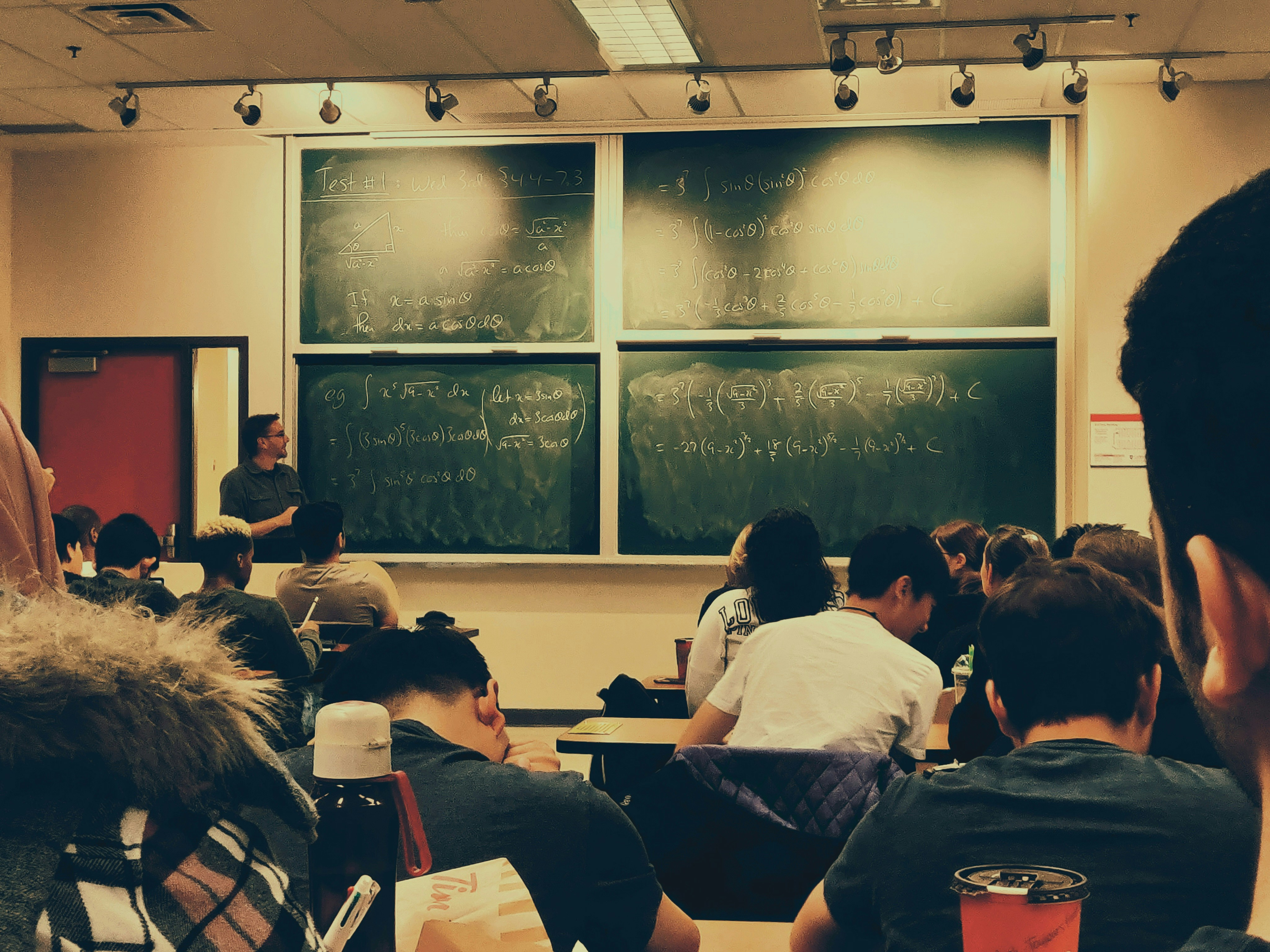## Before you go, check out these stories!

0The Knowledge of Arc Length & Limit of a Function by@james-johnson

# The Knowledge of Arc Length & Limit of a Function### @james-johnsonJames Johnson

I am a researcher and a technical content writer. A math teacher since 2007. I love travelling, Love

Background

We are usually familiar with the concept of length of anything by visualizing its straightness. In the beginning, it was widely believed among mathematicians that the length of any irregular curve could not be determined. This notion gradually faded away when Greek mathematician Archimedes opened up this debate again. His method of exhaustion gave the idea to measure volume and area beneath the circle, or any of its segments.

The definition of Arc Length can be formally stated that,

“The measure of the distance between two points along the curve is called its arc length.”

This curve can be the part of the circle or any other arbitrary curve. Arc lengths are expressed by “s” because the Latin word used for the length or magnitude is spatium. After the process of rectification, any curve can give a straight line segment with the same length as its curve's arc length.

Methods to Calculate

The formula of the arc measure is:

`` s=2πR[C/360]``

The above formula involves R, which is the radius, Pi (π), which has an approximate value of 3.142, and C, which is the central angle of the arc, measured in degrees.

2πR can measure the circumference of a circle; hence the formula of arc length reduces the previous method by the ratio of the central arc angle to a full angle of the circle, i.e., 360. These formulas are the base to calculate Arc length.

If the central angle is in radians, the formula is more straightforward:

``arc length = RC``

This will produce similar results in radian. One radian is approximately 57.3°. In the previous formula, the factor of 2π/360 converts the degrees into radians.

In term of Integrals

Determining the length of the curve is also a subject of consideration in infinitesimal Calculus, and the integral formula of measuring length was discovered by Hendrik van Heuraet and Pierre de Fermat in 1659.

The numerical integration of the arc length integral is usually very efficient. If a function f is defined on the interval [a, b], then the length L of the curve y=f(x) will be,

Suppose if you need to find the length of curve y= f(x) =x between the points a= 3 and b= 2.

In order to solve, we need a derivative of f(x),

``````  		dy/dx = d f(x)/dx = d(x)/dx
dy/dx = 1``````

And,

``````		       (dy/dx)2= 1
3
L = ∫2 √(1+1) dx
3
L = ∫2 √2 dx
L = √2 ∫(3-2)
L = √2``````

Thus, the arc length will be √2.

Limit of a Function

Historical Backdrop

Since the advent of civilization, Mathematics has been continuously responding to the unanswered questions of the universe. Sometimes, the answers may end up on concrete and absolute solutions, like in the case of Arithmetic and Geometry. Likewise, there are still some problems which have been responded to by inference and approximation, rather than a complete answer.

Why do we need Limits?

The concept of limit is one of the most classic examples of the latter category. It formally indicates the notion of arbitrary closeness. A limit exists when a variable quantity approaches a certain point to attain the most likely, and near-to-accurate result.

The theory of limits has its roots deeply stemmed into the properties of real numbers; how much two real numbers are closed to each other, there will always be another number in between these two real numbers. In Calculus, processes like differentiation and integration both are based on the theory of limits. Online limit calculators are meaningful to calculate limit functions.

What is a Function?

A function can be defined as a relation or expression involving one or more variables. Function usually contains ordered pairs, and when the elements of the ordered pairs belong to real numbers, the relationship can be expressed as an equation. Moreover,

“A function is a set of the ordered pairs for which the first and second elements of each pair should fixedly relate to one another.”

Limit and its Properties

Englishman Sir Issac Newton and German Gottfried Wilhelm von Leibniz both independently formulated the general principles and notations of calculus in the seventeenth century.

Typically, a Limit can be defined as,

``Lim x->a f(x)= L``

Which means that the limit of function f(x) is L as the value of x approaches to a.

The limit of a function has various properties, similar to the properties of real numbers.

Let suppose lim x->a f(x)= P, and lim x->a g(x)= Q then,

1. lim​ x->a (f(x) + g(x)) = P+Q
2. lim​ x->a (f(x) - g(x))  = P-Q
3. lim​ x->a (f(x)g(x))     = PQ
4. lim​ x->a (f(x)/g(x))    = P/Q (Q cannot be 0)
5. lim​ x->a (f(x))n          = Pn  (P, n should greater than 0)

One-Sided Limits

The limit can be either right-side or a left side limit of a function.

The right side limit of a function f,

``Lim x->a+ f(x) = L.``

The left-side limit of a function f is

``Lim x->a- f(x) = L``Join Hacker Noon